# What are equivalent fractions? The importance of showing, not telling, about equivalency.Share these ideas!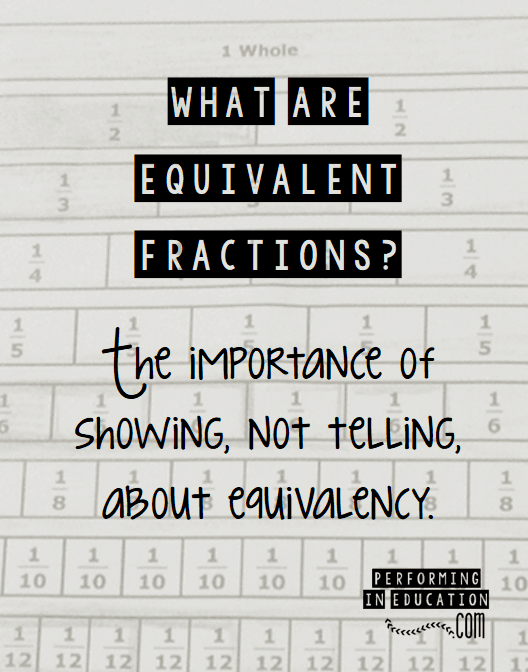#### We tell our students that equivalent means equal, but do they really know what equal means? Do they know that equal means the same amount, and not necessarily the same number representing that amount?

We started leading up to the correct understanding when we showed them models like these: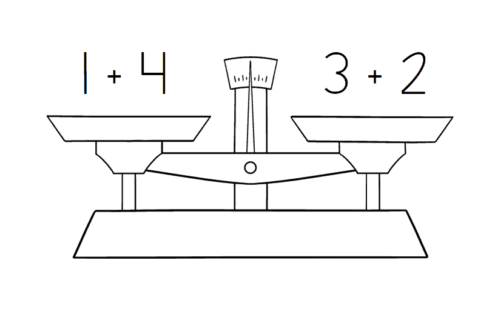And in measurement we tell them that 1 kg=1,000 g: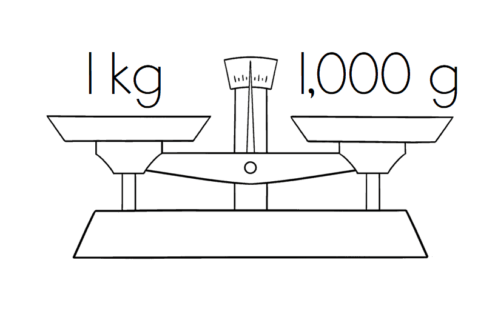Now we’re ready to show them that fractions can be equal too, even if the numbers in the numerator and denominator are different. But how can we explain why these are the same, when the numbers in one fraction seem so much bigger?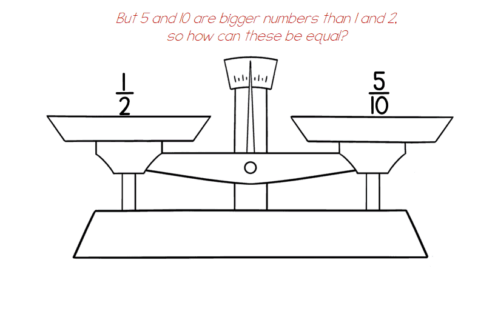### Equivalent Fractions

“Equivalent fractions are the same size.”
This is the definition I give my students the first day we begin discussing equivalent  fractions.
We then begin experimenting with our fraction cubes in groups. I give students the task to see how many towers they can build that are exactly the same size. When they find two (or three, or four…) that are the same size, they record the fraction on their paper.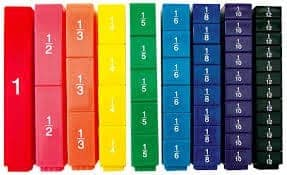Then I ask them, “Did you notice any differences in the equivalent fractions?”
Most students respond that there are a different number of pieces. This is the “aha!” moment for equivalent fractions. Equivalent fractions are the same size, even though they have a different number of pieces. This gives students the opportunity to build concrete evidence of fraction equivalency before we move on to more abstract problems.

### Fraction Families

I teach my students that equivalent fractions are a family. Equivalent fractions and “fraction families” are not only used to help us add and subtract fractions with unlike denominators, but they are a big part of understanding how to simplify fractions. In the activity below, I model finding equivalent fractions for each family, then circling the lowest term (the fraction with the largest pieces). This makes it very easy for students to visualize the size of each fraction and how they are related to each other.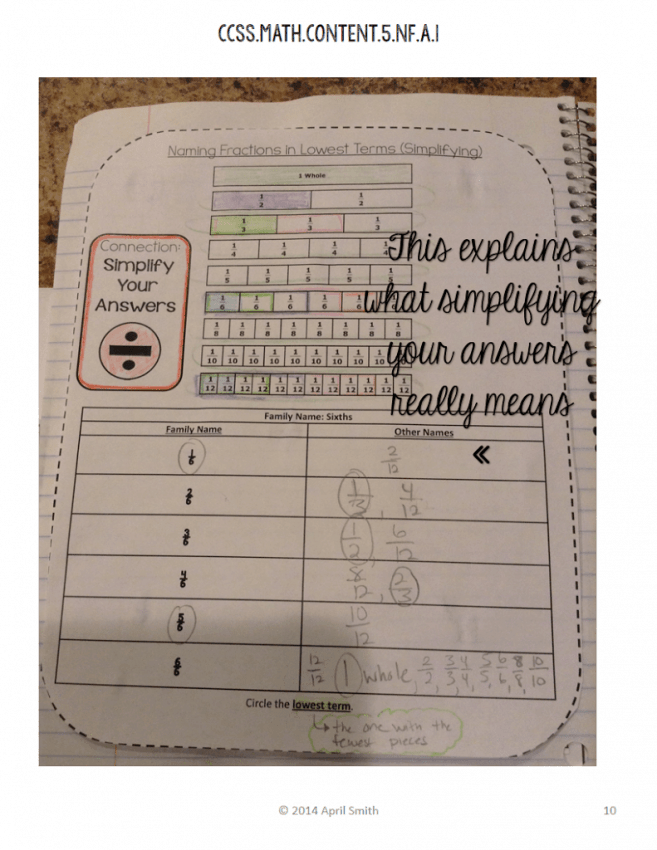Although teaching about fractions can be difficult, with an understanding of equivalency and LOTS of practice, your students will “get it”!Share these ideas!

Written by